# NCERT SOLUTIONS FOR CLASS 8  MATHS CHAPTER 2

Class 8 Chapter 2 Linear Equations in One Variable Solutions Maths Ncert are given.

First you study the textbook lesson Linear Equations in One Variable very well.

You should observe and practice the example problems and solutions given in the textbook.

Observe the given below solutions and try them in your own method.

NCERT class 8 maths solutions

# LINEAR EQUATIONS IN ONE VARIABLE SOLUTIONS

You can see the solutions of

Chapter 1. Rational numbers

Maths solutions class 10 real numbers

## CHAPTER 2 LINEAR EQUATIONS IN ONE VARIABLE PROBLEMS WITH SOLUTIONS

Exercise 2.3  = Exercise 2.1 now (for 2023 – 2024)

Exercise 2.5 = Exercise 2.2 now ( for 2023 – 2024)

EXERCISE 2.1 (Delated for 2023 – 2024)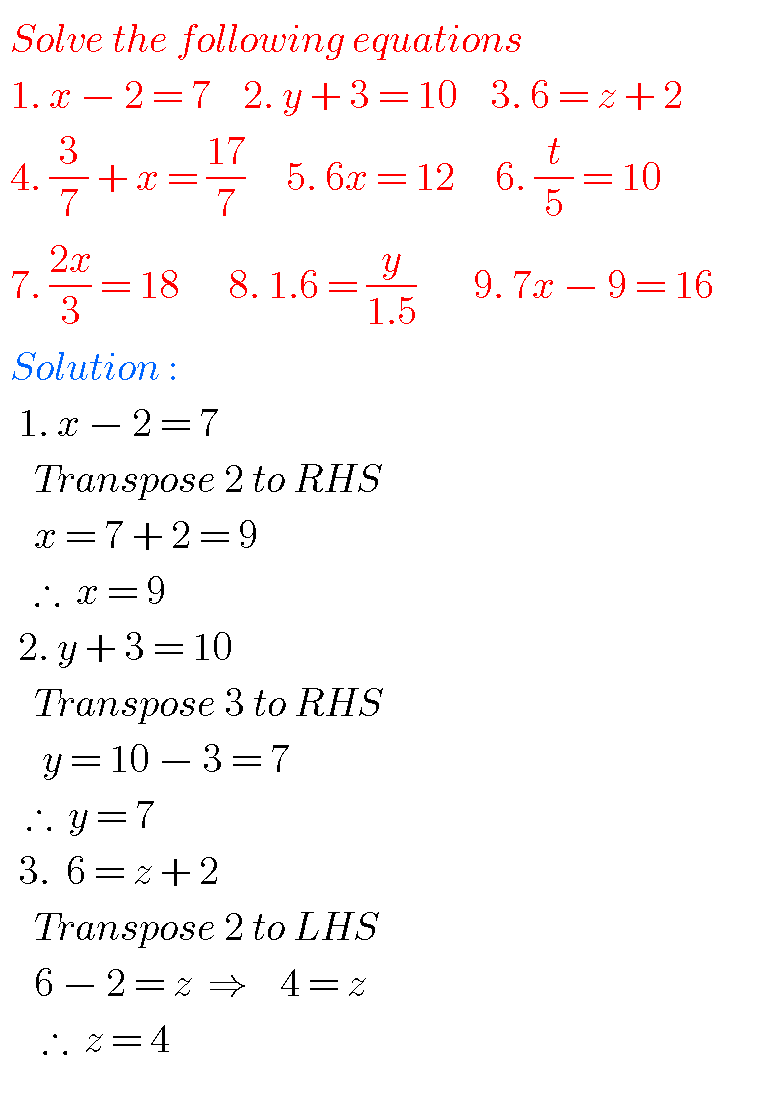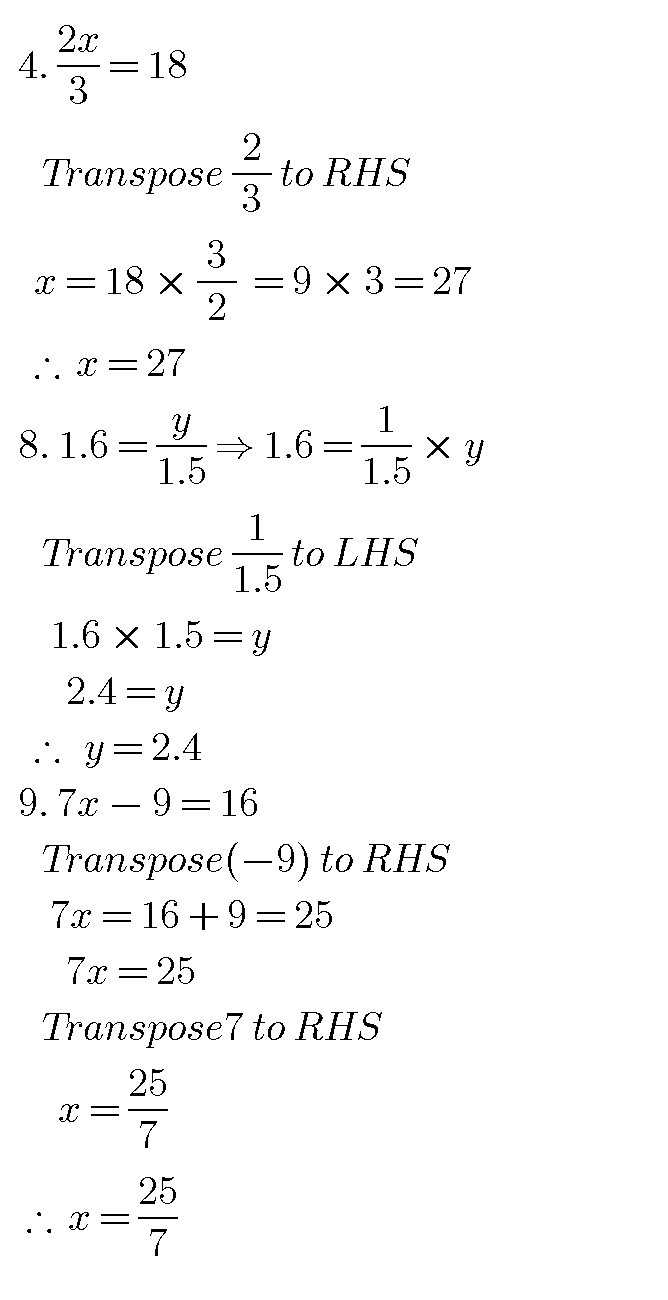### NCERT SOLUTIONS FOR CLASS 8 MATHS CHAPTER 2

EXERCISE 2. 2  (Delated for 2023 – 2024)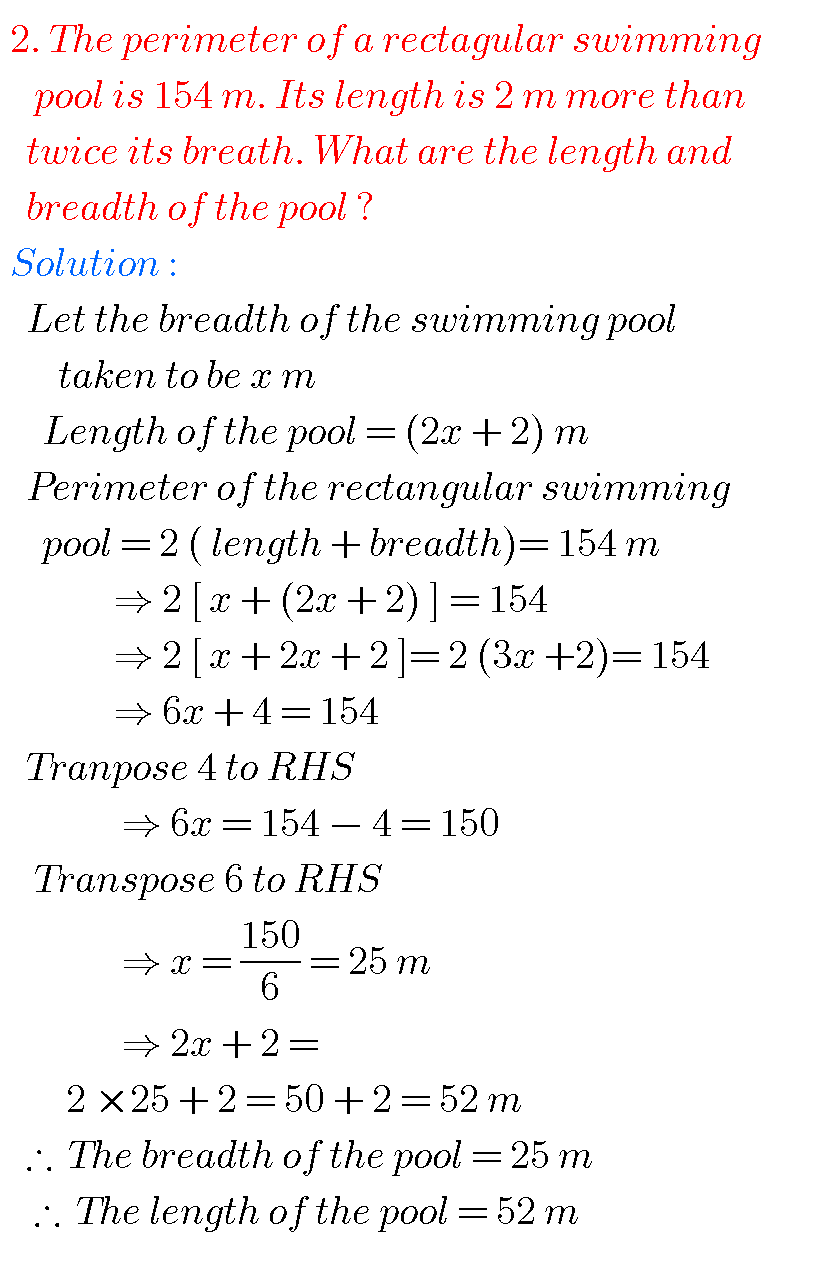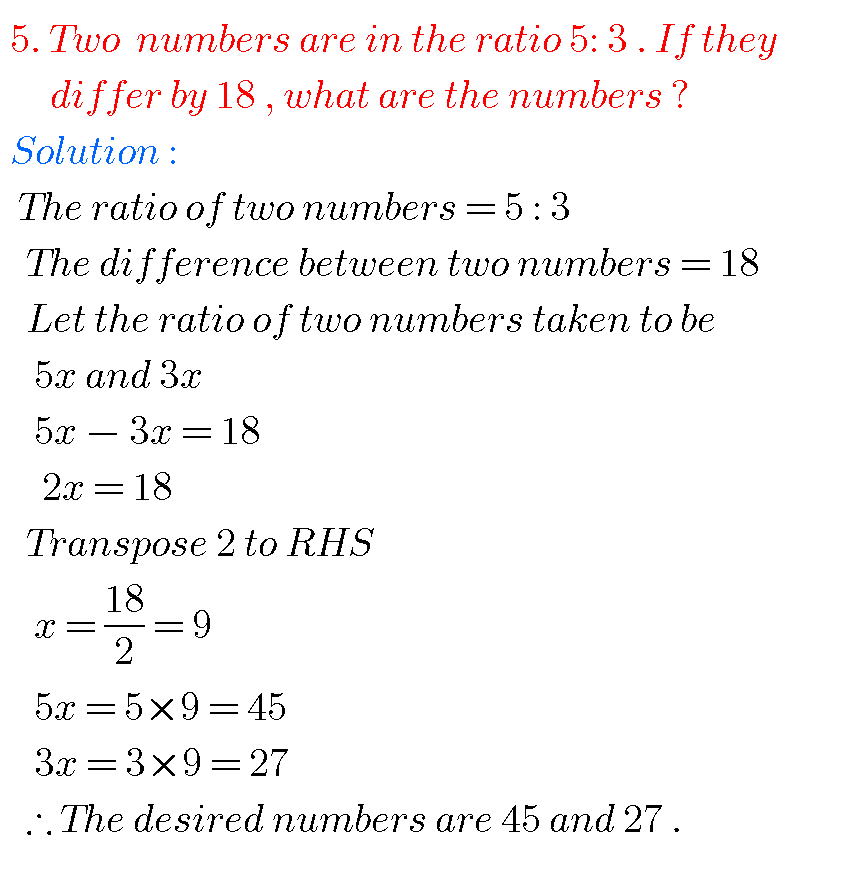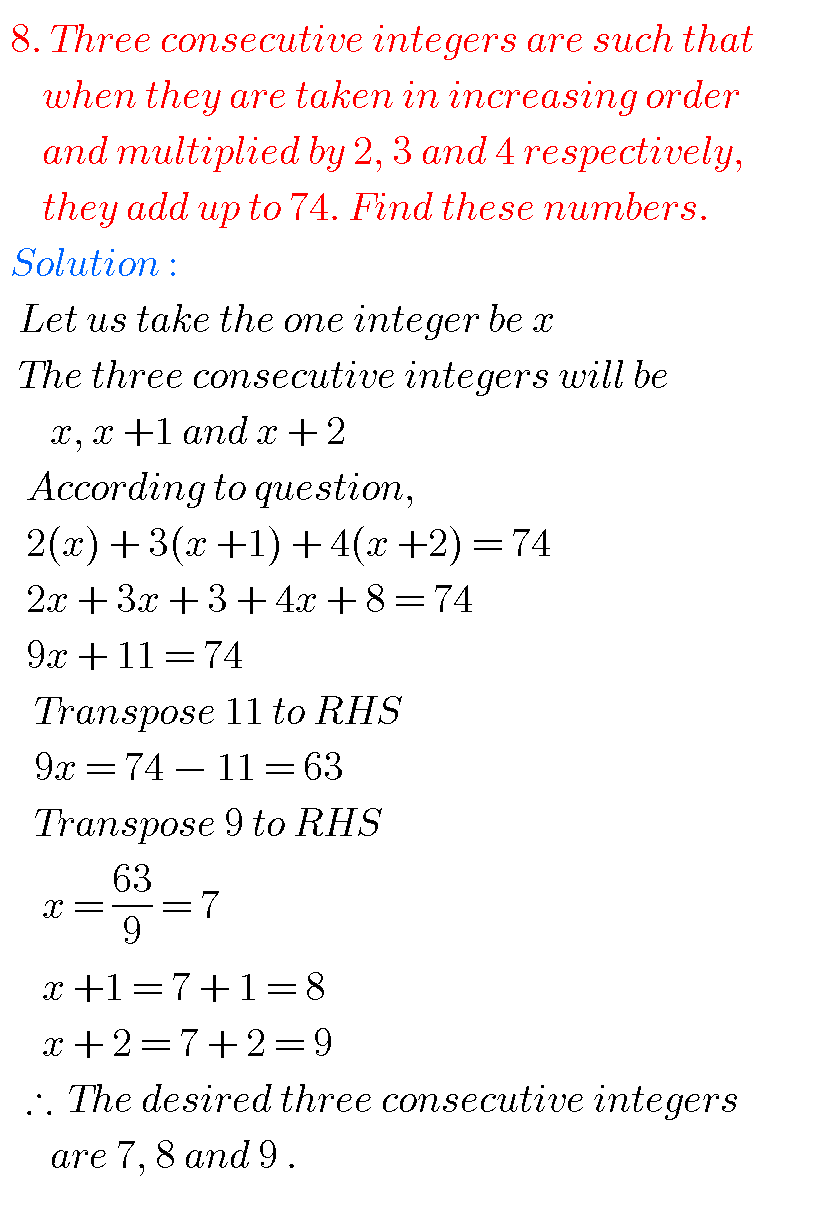###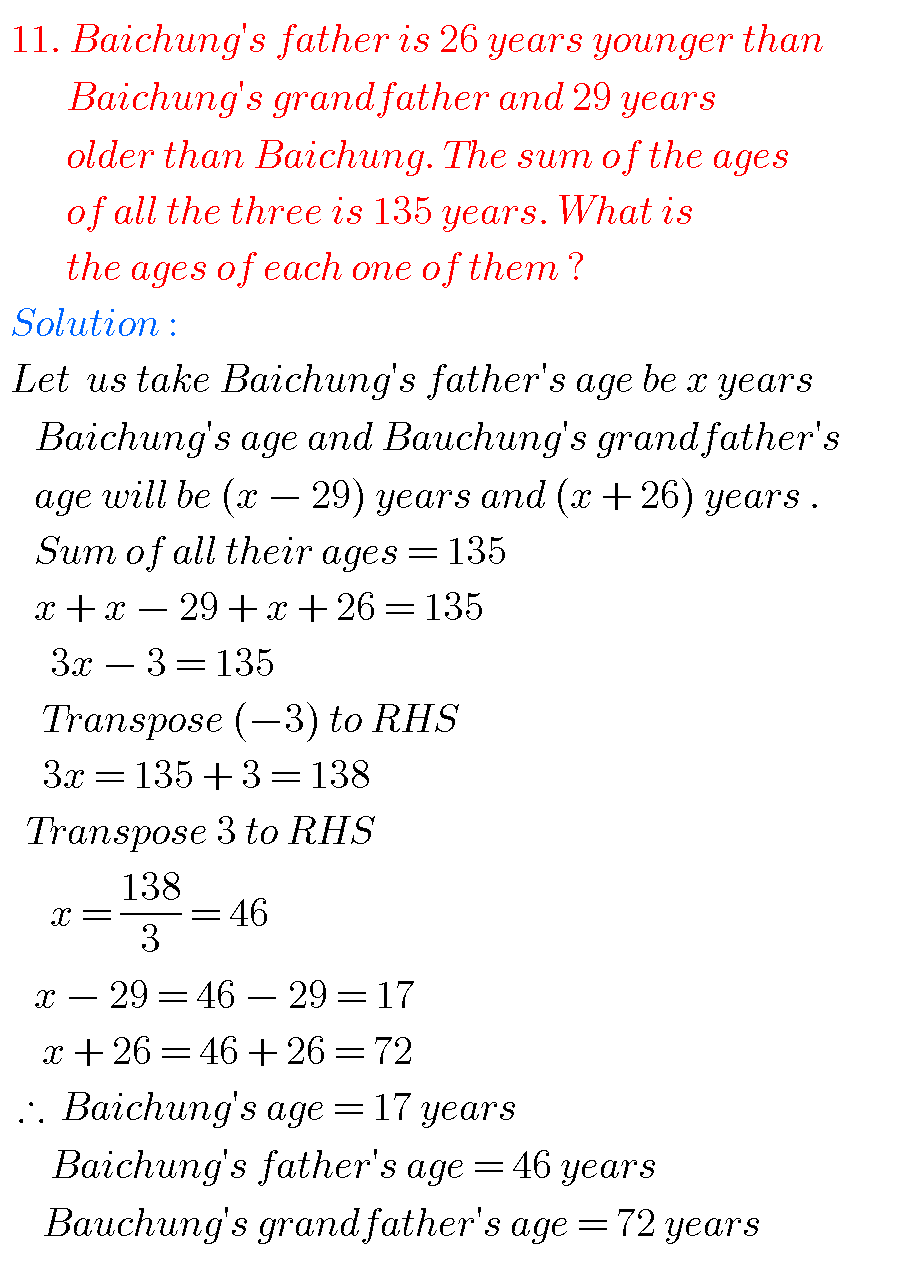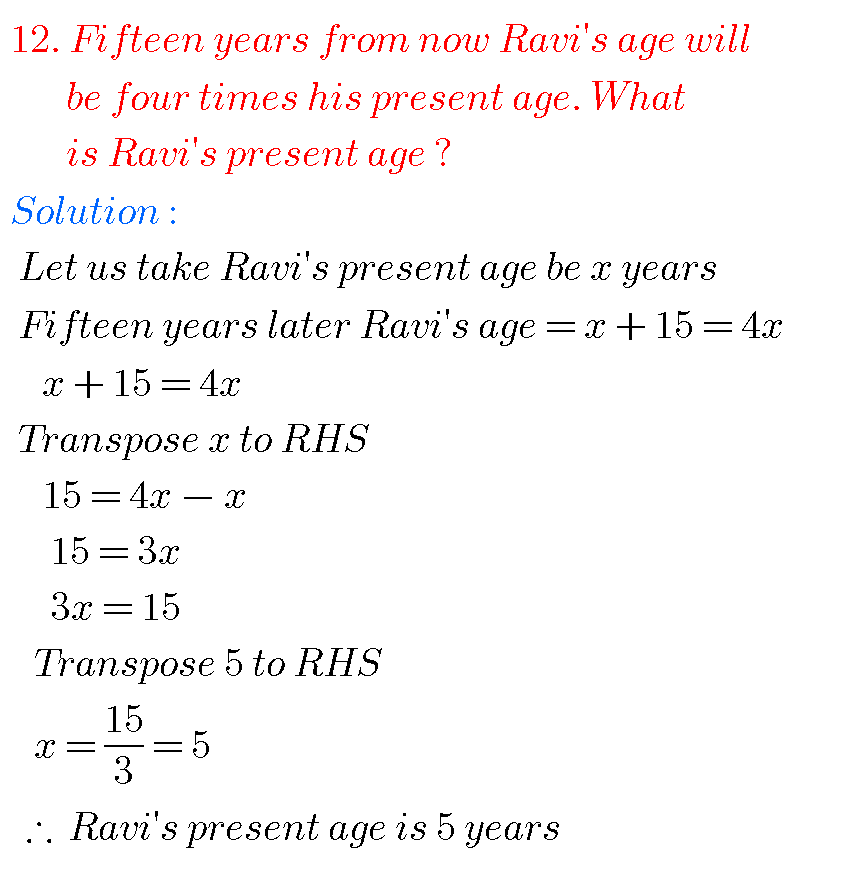###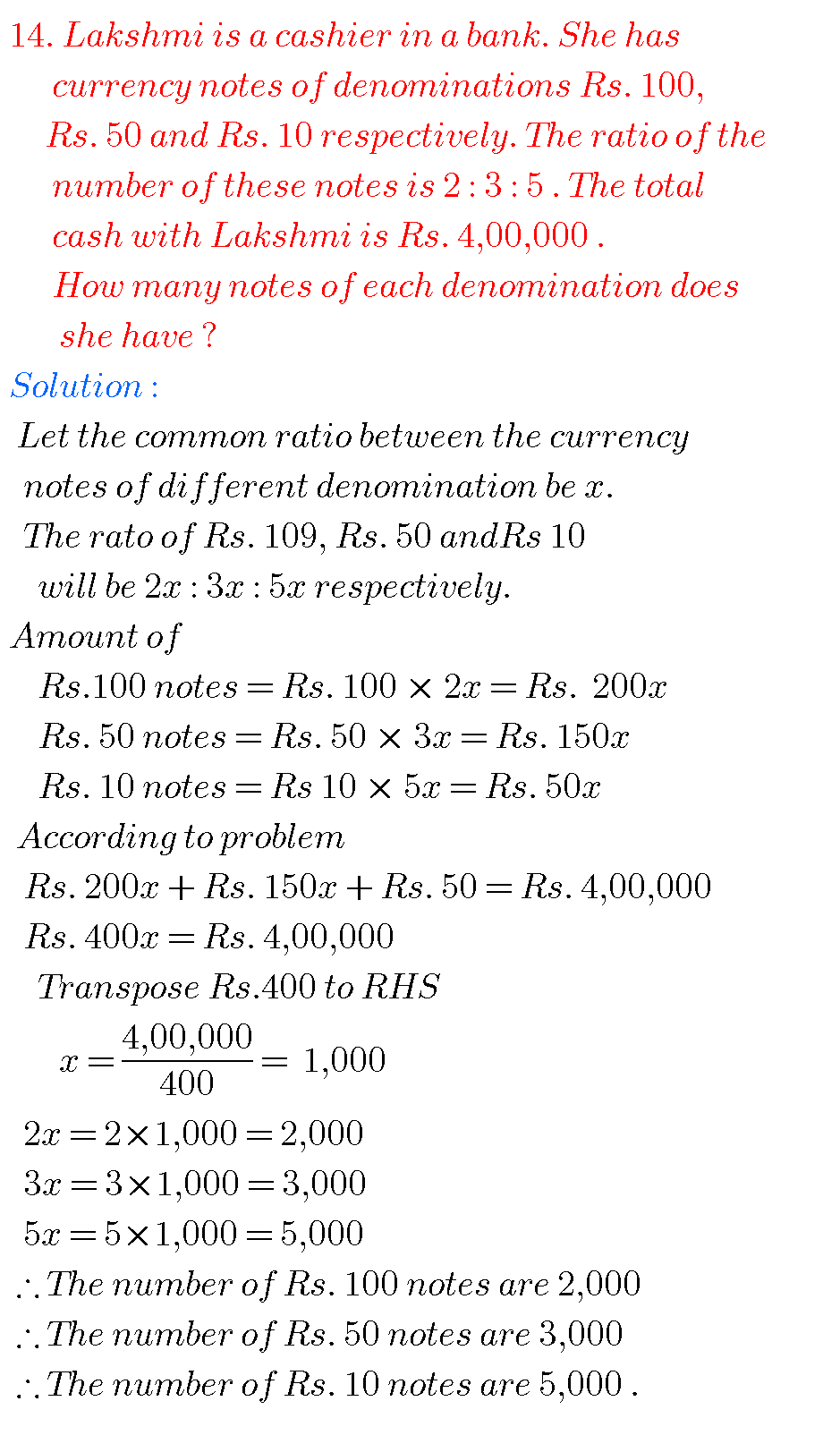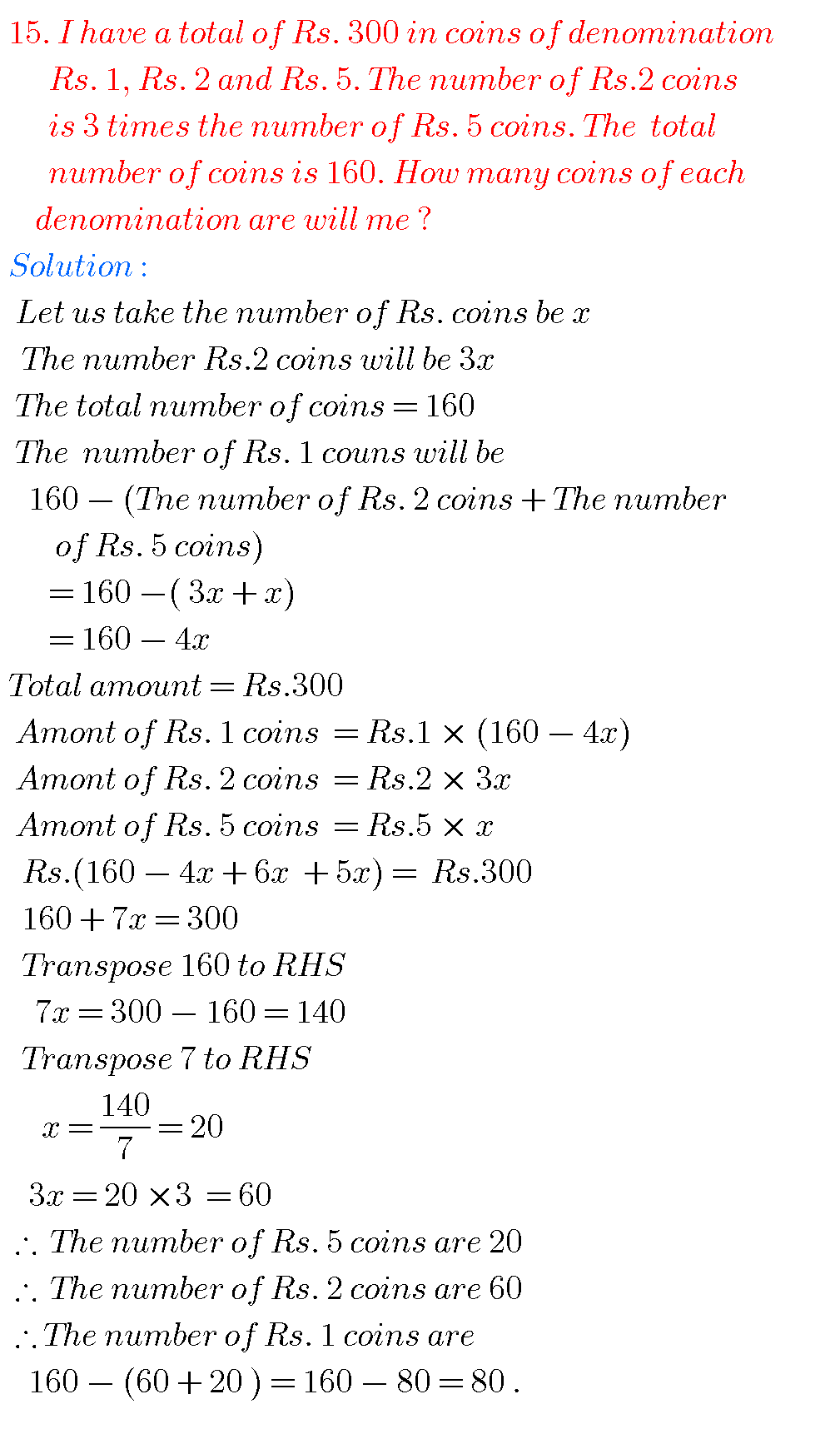### LINEAR EQUATIONS IN ONE VARIABLE SOLUTIONS NCERT class 8 maths

EXERCISE 2. 3 = Exercise 2.1(now for 2023 – 2024)##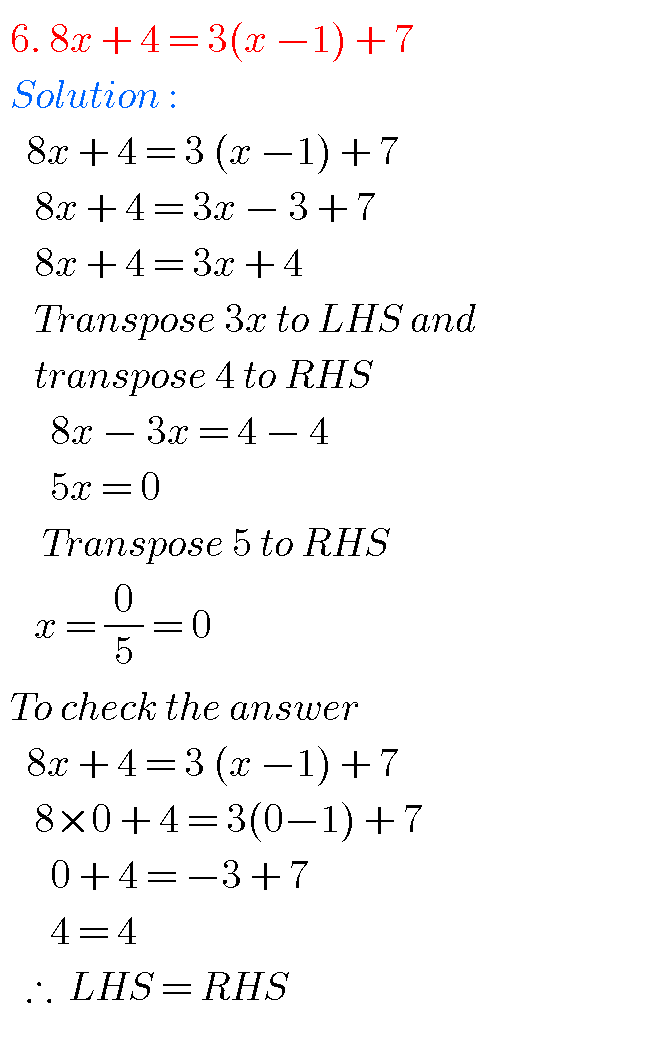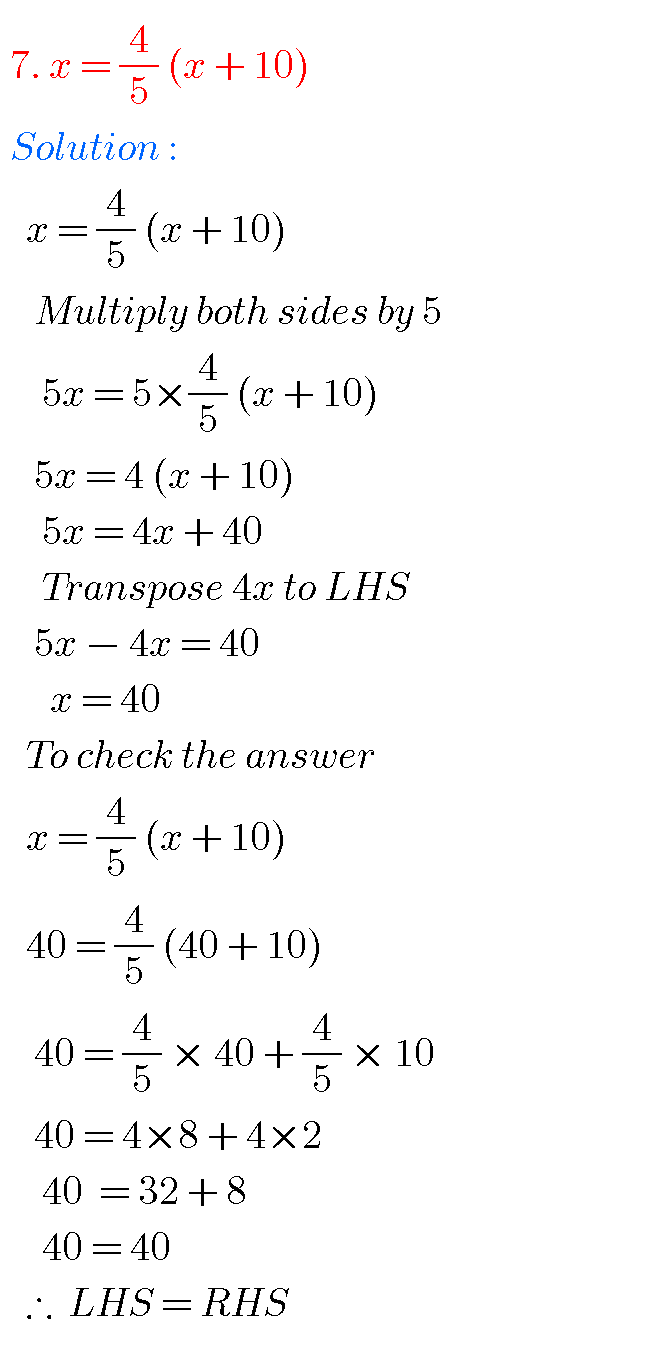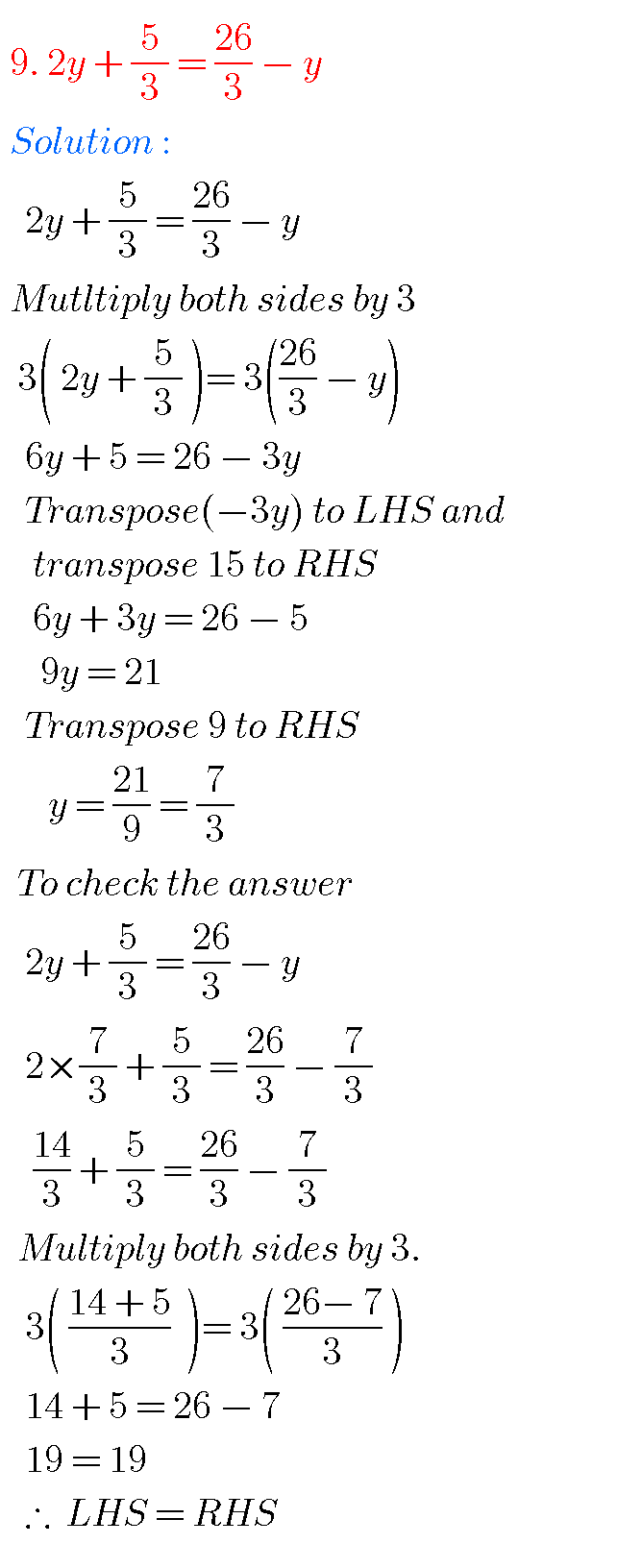## NCERT SOLUTIONS FOR CLASS 8 MATHS CHAPTER 2

EXERCISE 2. 4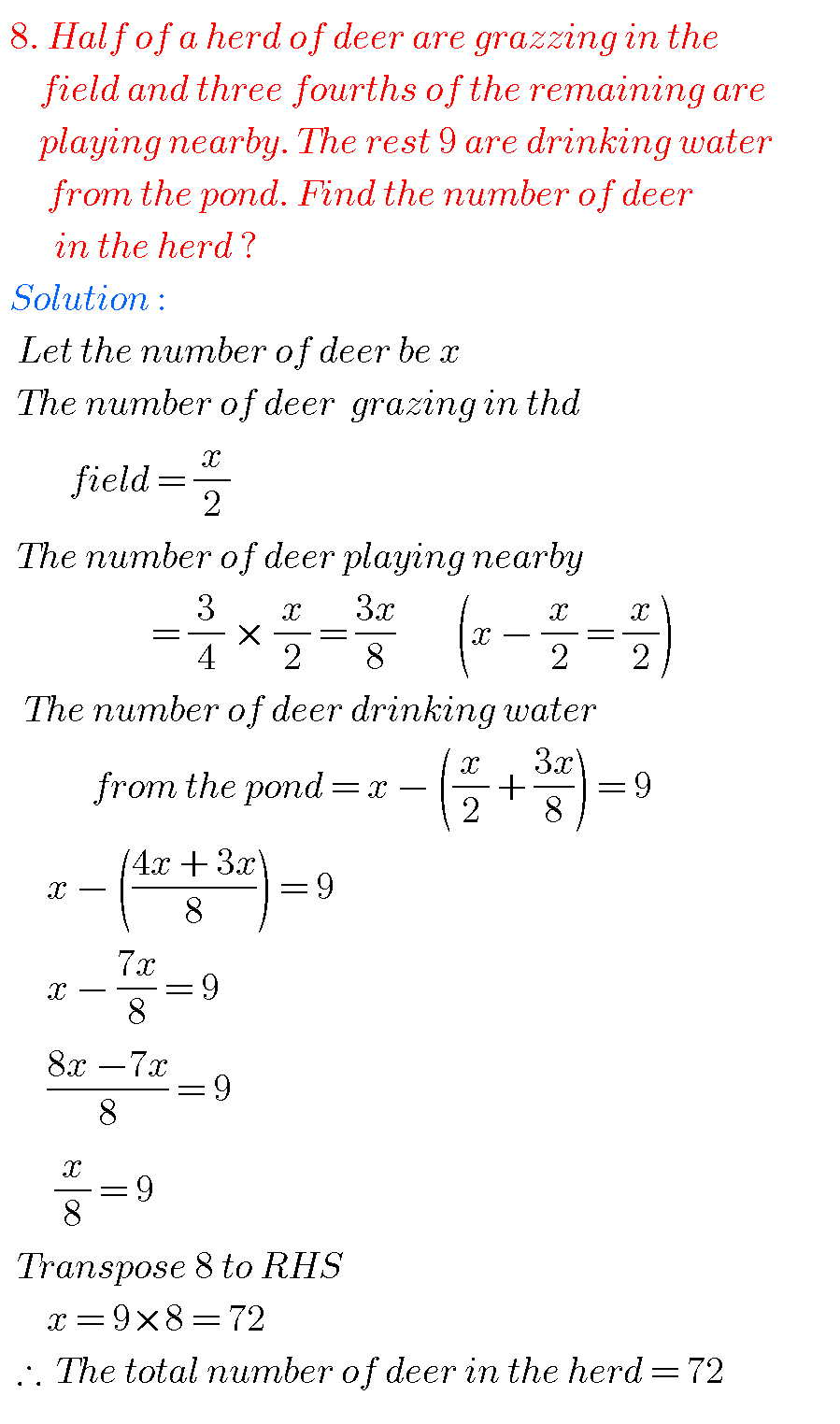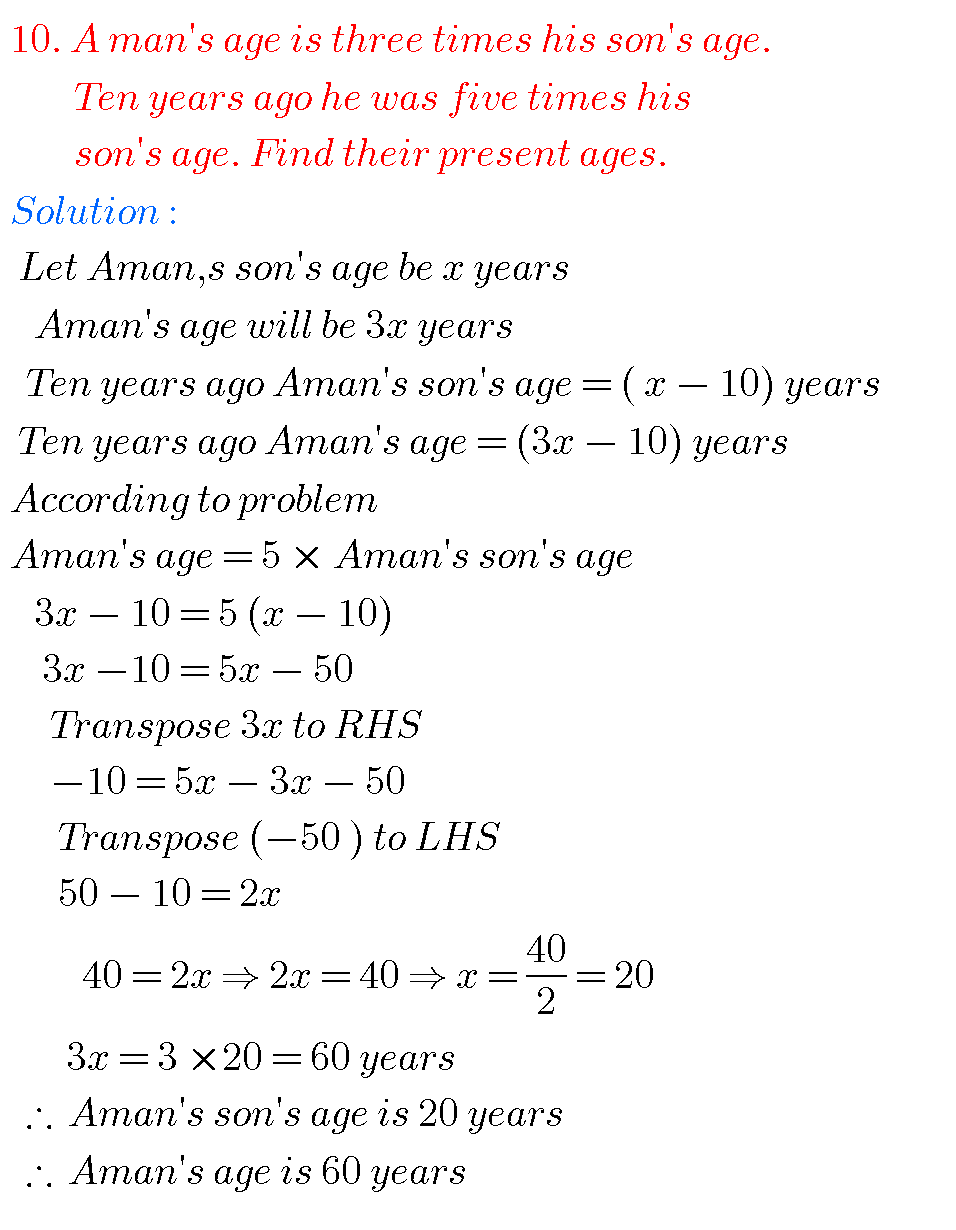EXERCISE 2.5 = Exercise 2.2 now( for 2023 – 2024)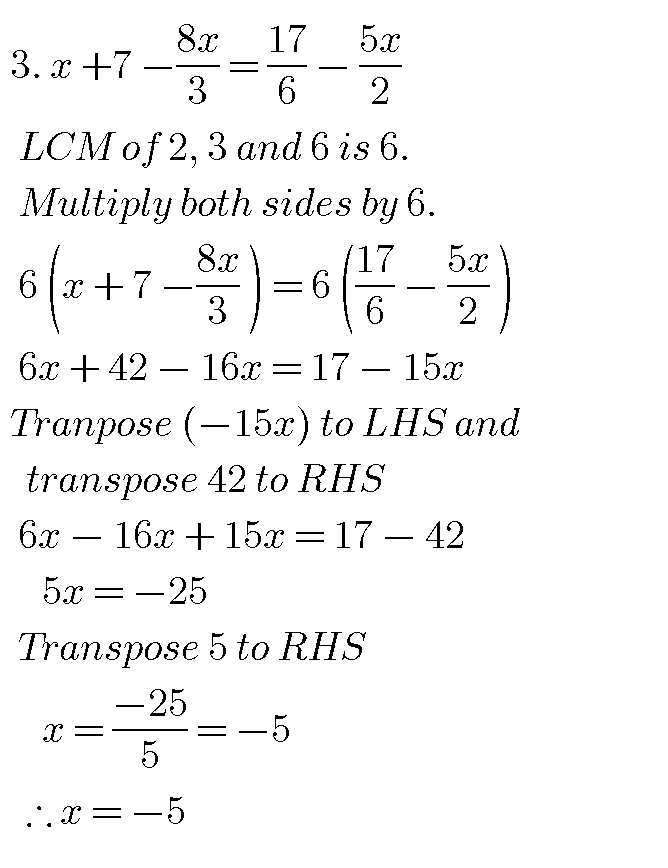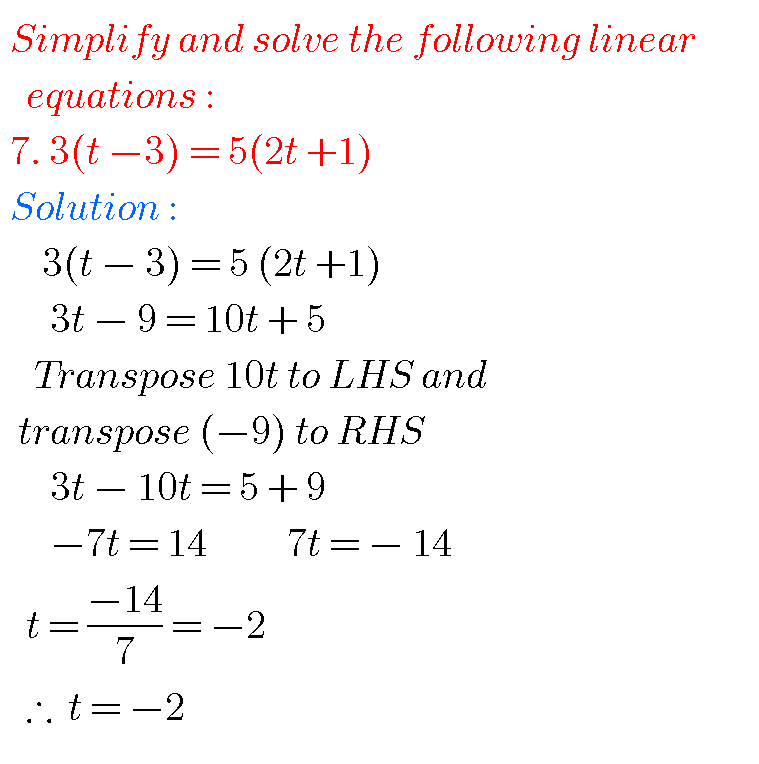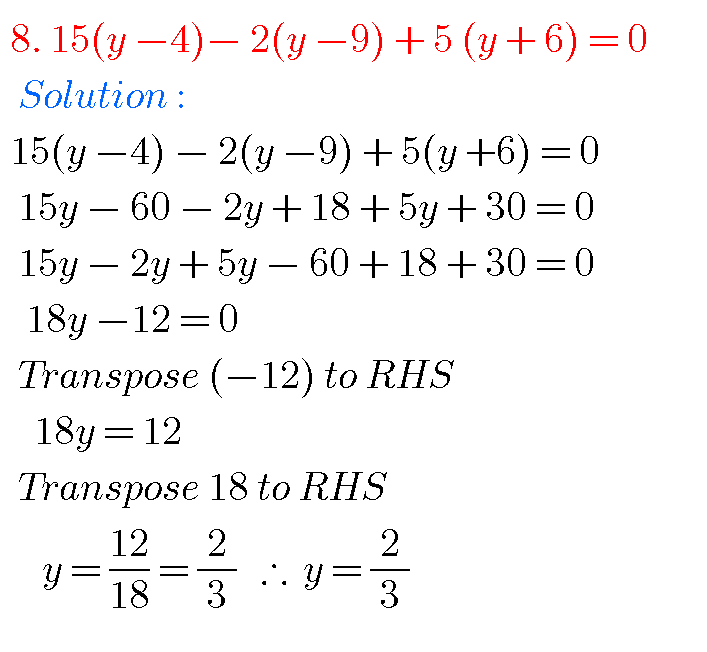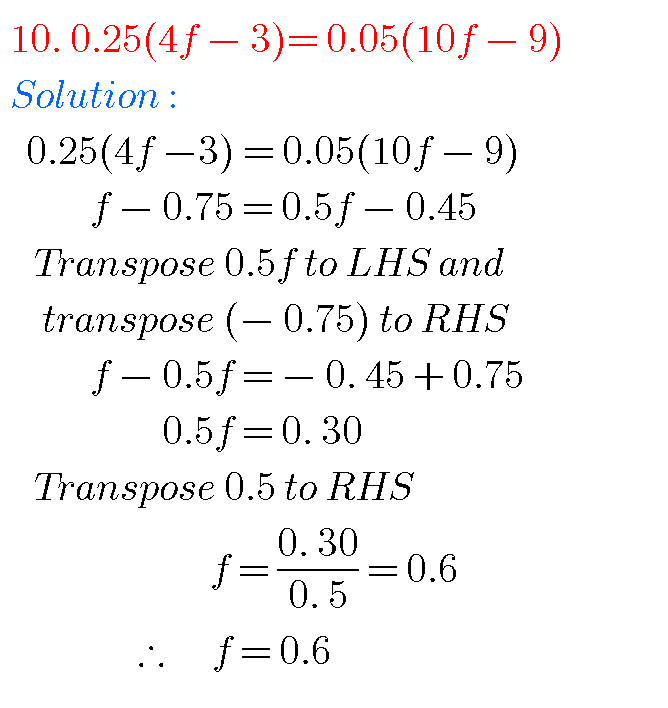EXERCISE 2.6 ( Delated for 2023 – 2024)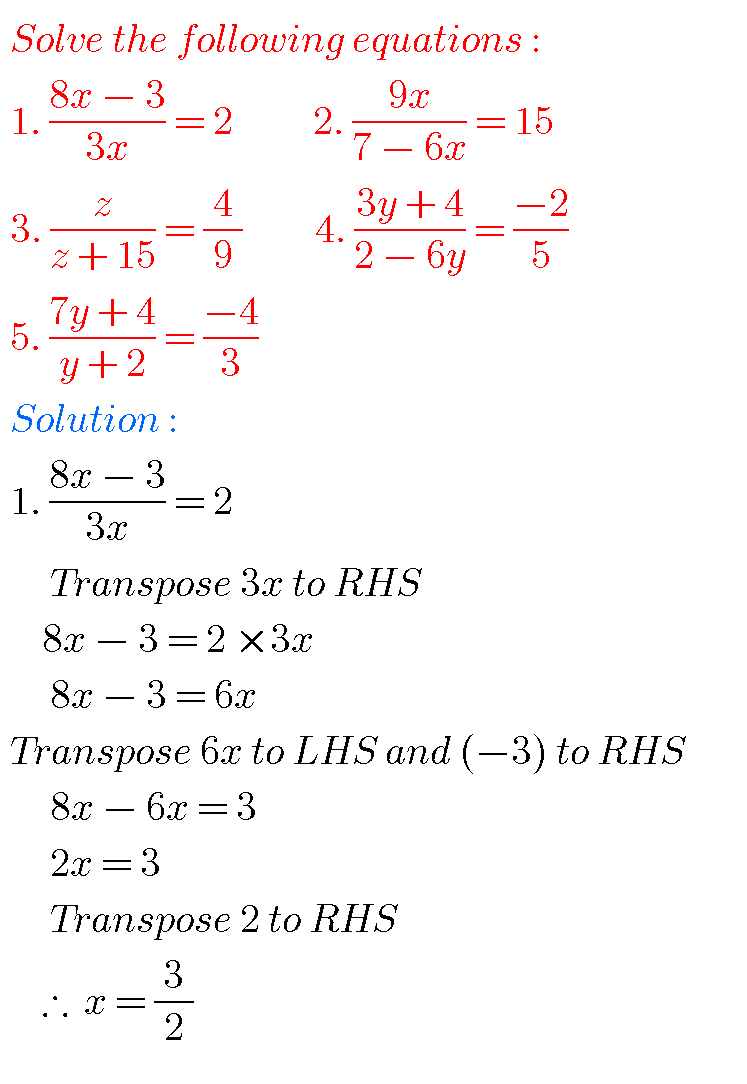NOTE : Observe the solutions of linear equations in one variable and try them in your own methods.

Ncert solutions for class 6 th chapter 1

Ncert solutions for class 7 th chapter 1

Ncert solutions for class 8

Chapter 1 rational numbers

Ncert solutions for maths class 6 some chapters

Ncert solutions for maths class 7 some chapters

Ncert solutions for maths class 8 some chapters

Ncert solutions for class 8 chapter 12

Intermediate functions solutions

Nios solutions for maths 311 book 1 Sets 1.1

### 2 thoughts on “Ncert solutions for class 8 maths chapter 2,Linear Equations in One Variable solutions”

1.Thank you Thank you So So Much

1.It’s too difficult😣